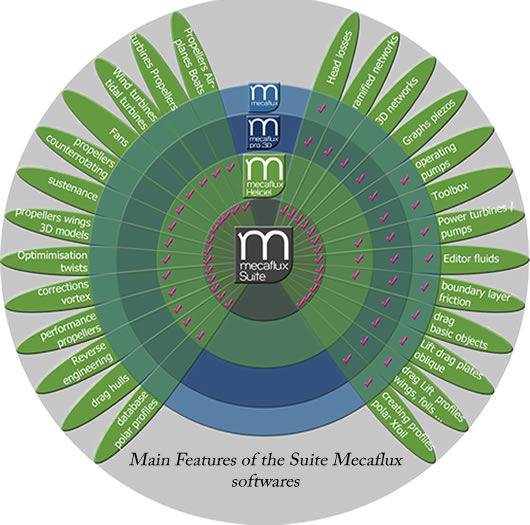Presentation Mecaflux softwares suite:
Mecaflux Suite Software comparative

Mecaflux Suite is a software suite to help mechanics calculations fluids. This software suite, tutorials and theoretical background online, provide a self-taught or knowledge building. The aim of this suite is to put within the reach of everyone the essential applications of fluid mechanics

The consulting firm found in this suite, the software tools needed to carry out specific projects dealing with fluids, the specialist will find applied media,and the student further information...

Here is an interactive chart summarizing the main functionalities of software suite Mecaflux::FunctionsChoice of fluid liquid or gas in database (Editor fluids) x x x x pipes Head losses calculation x x x Accessories Head losses calculation in x x x Inventory / cumulative load losses in a section composed of multiple elements x x x Calculation of complex ramified networks and loops x x Rapid design of networks: network modeling interface3D system with exclusive ergonomic layout with floating gate (Model filed mecaflux) x x Importing items and segment type "mecaflux standard" x x Edition graphics piezometric x x 3D animation modeling fluid network with variation in pressure x x Resolution pump operating point / pressure losses x x x Toolbox fluid mechanics (Bernoulli, emptying tank, hydrostatic pressure, converters, venturi, smoke pipes, sedimentation, thin layer, dynamic pressure viscometer, equivalent diameter, choice conduits) x x x Evaluation power turbine / pump x x x Calculations of basic objects Drag x x x Calculations Drag + boundary layer friction area x x x Calculation of lift plates oblique x x calcul de portance des ailes ou foils avec sélection profils aero/hydrodynamique x x x x Xfoil integration for creating profiles polar Edition x x x x Database profile interactive (selection, creation, performance profiles calculated aerodynamic / hydrodynamic) x x 3D modeling interface for propellers and wings x x Calculating Propellers capture (type tidal turbine, wind, Kaplan, propeller turbines) x x Calculation of propeller propulsion (type aircraft, helicopters, boats, ventilators, ventilation, pumps, propellers) x x Calculation of performance (power, torque, attacks) according to the specifications of the project x x Incorporating corrections effects of losses at tip blades and wings (vortex theory) x x Calculation of optimum propeller twists (Theory blade elements with Prandtl and Glauert corrections) x x Generation of 3D file formats for export igs in CAD ​​(eg Solidworks) surface BSplines entity igs 128 (smoothing surfaces) x x Editing curves performance test based on rotational speed range or velocity fluid x x Reverse Engineering: Setting a propeller existing by entry of the pitche and profiles) x x Contrarotating propellers: Calculation of the propeller by insertion downstream of axial and tangential flow induced by the upstream propeller) x x Calculation of hull forms drag modeled by selecting profiles x x